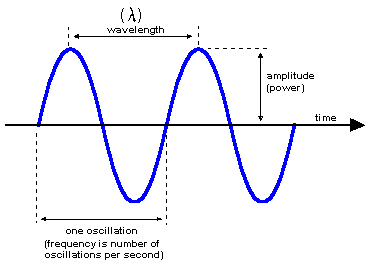# Definition: frequency

The number of oscillations (vibrations) in one second. Frequency is measured in Hertz (Hz), which is the same as "oscillations per second" or "cycles per second." For example, the alternating current in a wall outlet in the U.S. and Canada is 60Hz. Electromagnetic radiation is measured in kiloHertz (kHz), megahertz (MHz) and gigahertz (GHz). See wavelength, frequency response, audio, carrier and space/time.Frequency
The frequency is the number of oscillations per second. The higher the frequency (the closer the ripples would be in this diagram) and the shorter the wavelength.